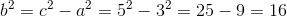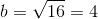# SSAT Middle Level Math : How to find a triangle on a coordinate plane

## Example Questions

### Example Question #1 : How To Find A Triangle On A Coordinate Plane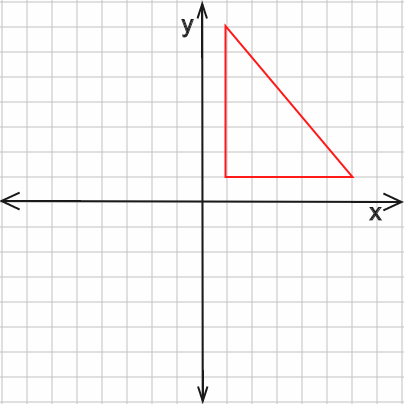Find the area of the above triangle, given that it has a height of 12 and a base of 10.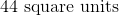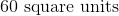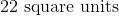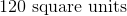Explanation:

Because this is a right triangle, the area formula is simply: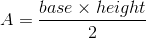Thus, the solution is: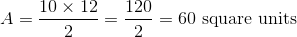### Example Question #2 : How To Find A Triangle On A Coordinate Plane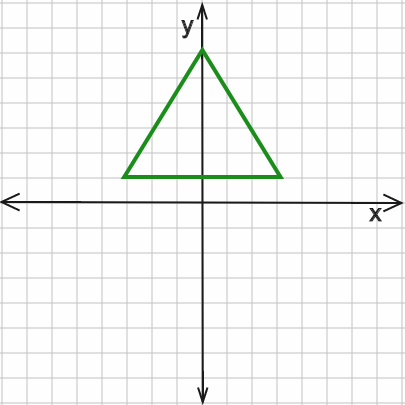Given the above triangle is an equilateral triangle, find the perimeter in units as drawn in the coordinate system.Explanation:

Using the coordinate system, one can see the base of the triangle is 6 units in length. Since it is an equilateral triangle, the other two sides must also be 6 units each in length. Therefore the perimeter is: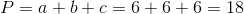### Example Question #3 : How To Find A Triangle On A Coordinate PlaneThe isosceles triangle shown above has a perimeter of 22 and base of 6. Find the lengths of the left and right sides, respectively. Assume no other side has a length of 6.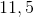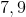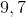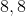Explanation:

With a perimeter of 22 and base of 6, this means the other two sides must add up to: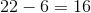Because an isosceles triangle must have two sides equal in length and we know from the problem that no other side equals 6, the two remaining sides must be equal to each other.

Thus, to be equal to each other and also add up to 16, each side must be 8 units in length.

### Example Question #4 : How To Find A Triangle On A Coordinate PlaneGiven the above triangle has a base of 5 and height of 6, what is the perimeter of the triangle?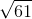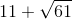Explanation:

First, use the Pythagorean Theorem to find the length of the hypotenuse:whereandare 5 and 6, respectively, andis the hypotenuse.

Thus,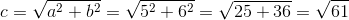Finally, the perimeter is the sum of the sides of the triangle or: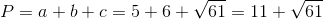### Example Question #5 : How To Find A Triangle On A Coordinate Plane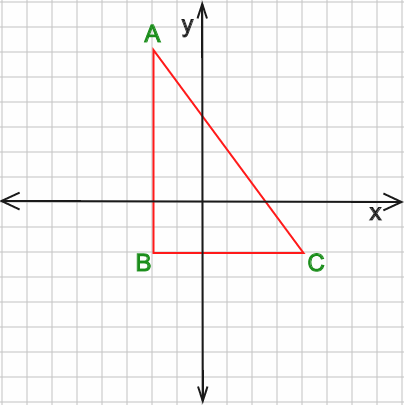Given triangle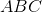, whereis at point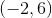andis at point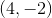, find the area.Explanation:

To find the area of this triangle, we first need to determine the length of sides AB and BC. First, point B shares the same x-coordinate as point A and the same y-coordinate as point C. Thus, B must be located at point (-2,-2).

The length of side AB must then be: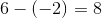and the length of side BC: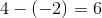Using the area formula,we can find the area using the base (side BC) and height (side AB):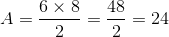### Example Question #6 : How To Find A Triangle On A Coordinate PlaneGiven triangle, where side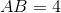and side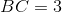, find the perimeter.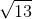Explanation:

Use the Pythagorean Theorem to find the length of side AC: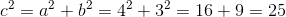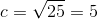Then, the perimeter is simply the sum of all three sides: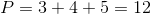### Example Question #7 : How To Find A Triangle On A Coordinate PlaneThe above triangle has base 6 and height 4. Find the perimeter.Explanation:

Because the y-axis bisects the base, we can divide the triangle into two, equal right triangles. The base of the right triangle is thus half that of the larger triangle, or 3. The height is still 4. To find the hypotenuse, use the Pythagorean Theorem: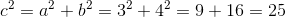Thus, we now know the base as given in the problem and each of the other two sides (which are also the hypotenuses of the right triangles).

Therefore, the perimeter is: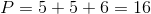### Example Question #8 : How To Find A Triangle On A Coordinate PlaneGiven a height ofunits and base ofunits, find the area of the triangle shown above.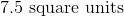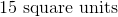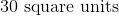Explanation:

The area of any triangle is calculated by the formula: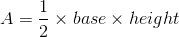Thus, the area of this triangle is: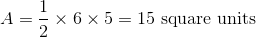### Example Question #9 : How To Find A Triangle On A Coordinate Plane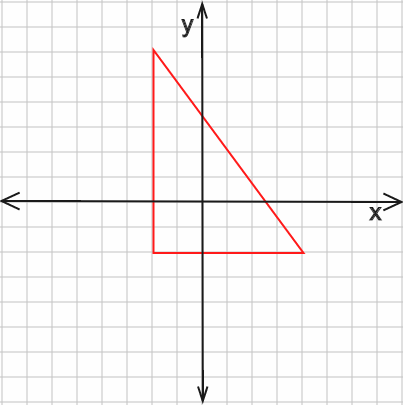Given the above triangle has a base ofand hypotenuse of, find the height of the triangle.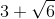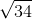Explanation:

Use the Pythagorean Theorem,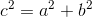whereis the hypotenuse,is the base, andis the height.

Rearranging to solve for the height,, yields: